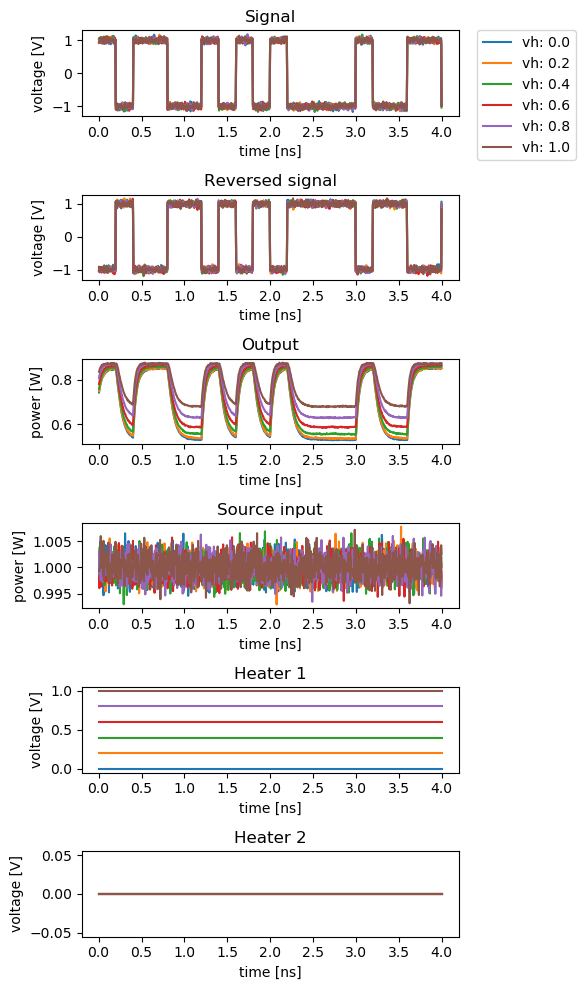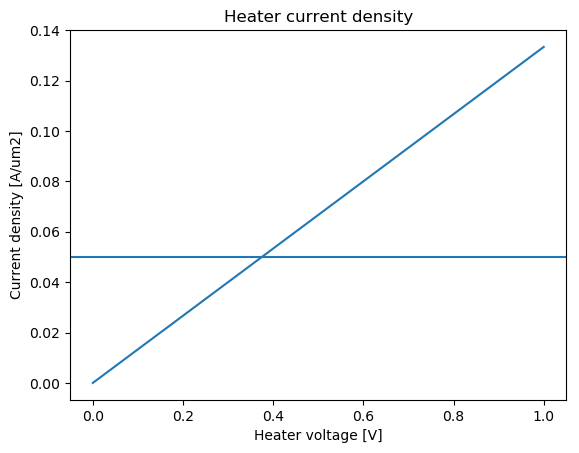# Mach-Zehnder modulator¶

## MZMModulator¶

SiFab contains a Mach-Zehnder modulator (MZM). This device is based on a Mach-Zehnder interferometer, where the light is first split in two branches and is then recombined by interference. On each branch, a phase shifter is placed to control the relative phase shift of the light on the two branches. This allows to modulate the phase in order to achieve constructive or destructive interference, which results in an amplitude modulation.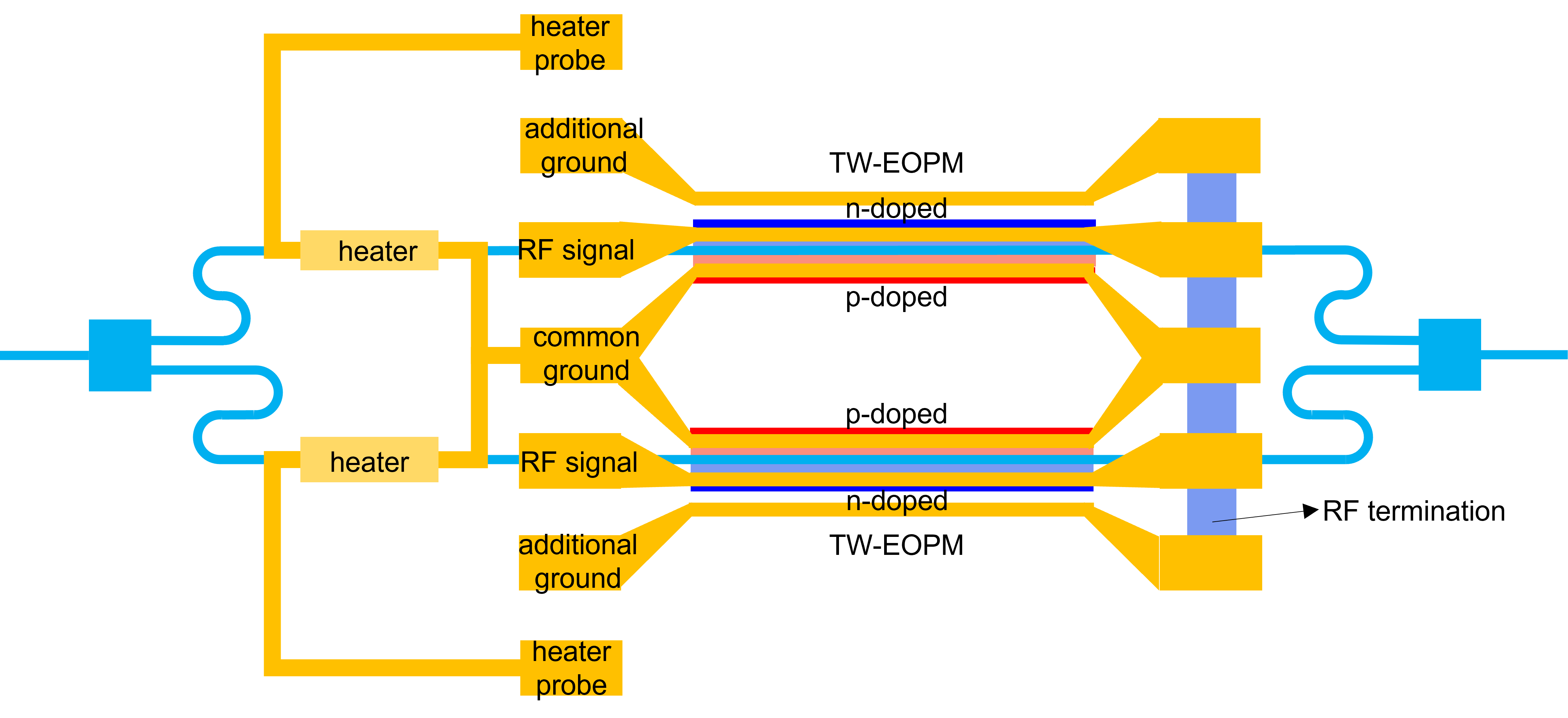The light is first split in two using a splitter, which can be provided through the splitter property. A taper is used to transition from the waveguide mode in the splitter waveguide to the waveguide mode in the phase shifters, which are placed on each arm. The phase shifter are controlled by the phaseshifter property.

The distance between the splitter and the phase shifter on each arm is controlled through the property spacing_x.

The two arms of the MZI of the MZM are designed to have a $$\pi / 2$$-length difference at center_wavelength, so that the modulator has maximum extinction ratio at center_wavelength, which is the wavelength of operation. Both arms are equipped with waveguide heaters controlled by the property heater to make sure that the offset of $$\pi/2$$ can be maintained over different operation conditions.The heaters are connected using a M1M2 wire with width heater_elpath_width.

The modulator works in a differential push-pull configuration driven by a single GSGSG line with a contact pitch of rf_pitch, length rf_pad_length and width rf_pad_width. The width of the signals lines is rf_signal_width, while the width of the ground lines is rf_ground_width.

The GSGSG lines are terminated by a load resitance resistance located at a distance given by resistance_spacing after the end of the transmission line.

### Layout¶

The following example demonstrates how to instantiate an MZM and export the layout to GDSII.

from si_fab import all as pdk

# Phase Shifter
ps = pdk.PhaseShifterWaveguide(length=1000.0,
trace_template=pdk.RWG450(),
junction_offset=-0.1)

heater = pdk.HeatedWaveguide(name="heater")
heater.Layout(shape=[(0.0, 0.0), (100.0, 0.0)])
# Modulator
mzm = pdk.MZMModulator(phaseshifter=ps,
heater=heater,
spacing_x=40,
rf_pitch=100.0,
rf_signal_width=5.0,
rf_ground_width=20.0,
resistor_spacing=15.0,
heater_elpath_width=5.0)

mzm_lv = mzm.Layout()
mzm_lv.write_gdsii("mzm.gds")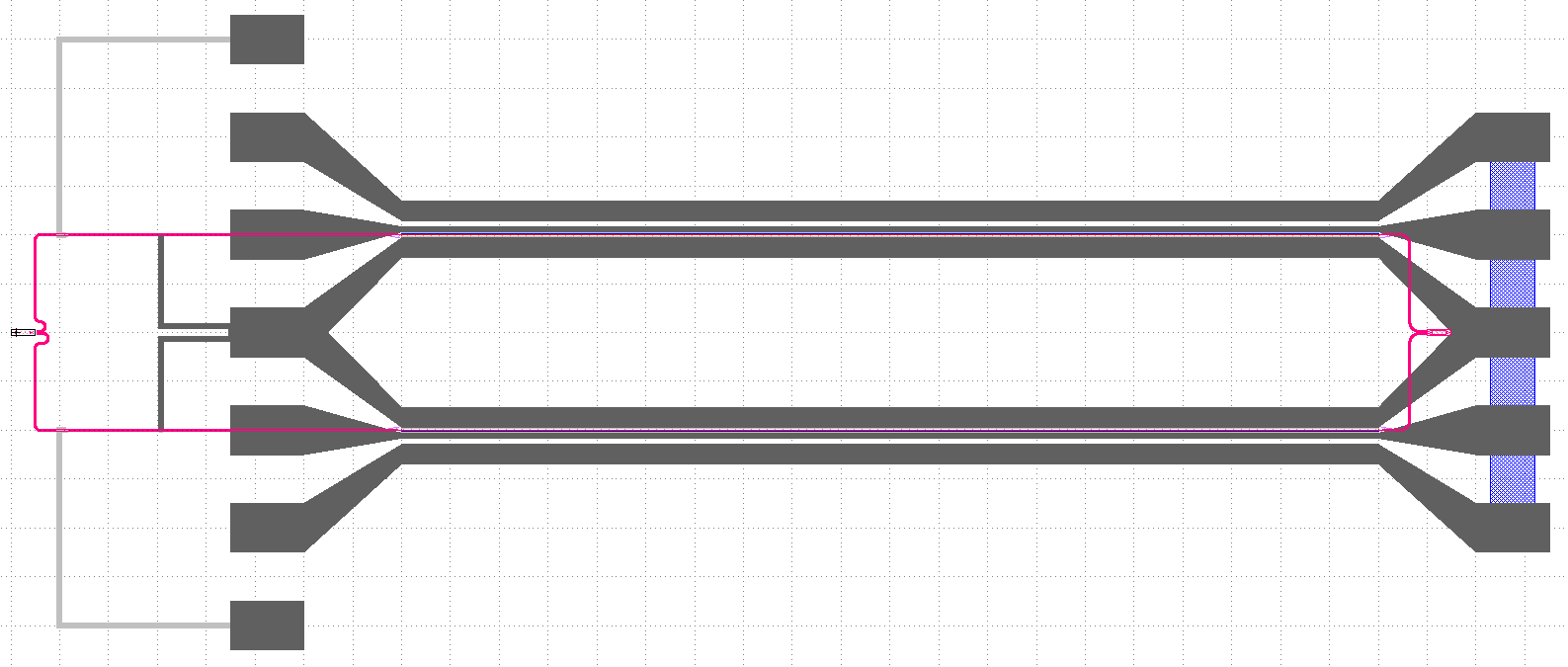#### Cross section¶

The following example plots the cross-section of the MZM.

from si_fab import all as pdk
from ipkiss3 import all as i3

# Phase Shifter
ps = pdk.PhaseShifterWaveguide(length=2.0,
trace_template=pdk.RWG450(),
junction_offset=-0.1)
# Modulator
mzm = pdk.MZMModulator(phaseshifter=ps,
spacing_x=40,
rf_pitch=25.0,
rf_signal_width=5.0,
rf_ground_width=10.0,
resistor_spacing=15.0,
heater_elpath_width=5.0)

mzm_lv = mzm.Layout()
xs = mzm_lv.cross_section(cross_section_path=i3.Shape([(1.0, -35.0), (1.0, 35.0)]))  # Can be slow
fig = xs.visualize(show=False)
fig.savefig("mod_cross_section.png", bbox_inches='tight')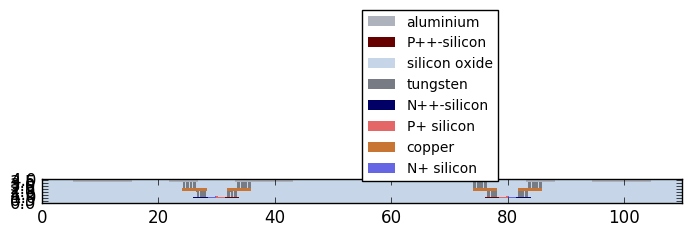#### Model and simulation recipes¶

The circuit model of the MZM is a hierarchical model that is built on the individual models of the following components:

• Phase shifter
• Splitter
• Taper
• Heater
• Waveguides

A simulation recipes is used to set up a test bench, which uses a PRBS signal as a function of the following parameters:

• cell: PCell of the MZM to simulate.
• mod_amplitude: Amplitude of the modulator.
• mod_noise: Amplitude of the noise on the modulator signal.
• opt_amplitude: Amplitude of the input signal.
• opt_noise: Amplitude of the noise at the optical input.
• v_heater1: Voltage over the first heater [V].
• v_heater2: Voltage over the second heater [V].
• bitrate: Bit rate of the signal.
• n_bytes: Number of bytes of the simulations.
• steps_per_bit: Number of time steps per bit.
• center_wavelength: Center wavelength of the optical carrier.
• simulate_sources: Simulate the sources.
• simulate_circuit: Simulate the circuit.
• debug: Debug mode.
• seed: Seed of the random number generation.

The test bench returns a dictionary of signals that can be used to do post-processing and plotting.

from si_fab import all as pdk
from si_fab.components.modulator.mzm.simulate.simulate import simulate_modulation
import pylab as plt
import numpy as np
import os

# Phase Shifter
name = "simpler_simulation"
results_array = []
length = 1000.0
ps = pdk.PhaseShifterWaveguide(length=length,
trace_template=pdk.RWG450(),
junction_offset=-0.1)
vpi_lpi = 1.2
Cl = 1.1e-15  # F/um
R = 50
tau = ps.length * Cl * R
ps.CircuitModel(vpi_lpi=vpi_lpi)

heater = pdk.HeatedWaveguide(name="heater")
heater.Layout(shape=[(0.0, 0.0), (100.0, 0.0)])

mzm = pdk.MZMModulator(phaseshifter=ps,
heater=heater)

results = simulate_modulation(cell=mzm,
mod_amplitude=1.0,
mod_noise=0.15,
opt_amplitude=1.0,
opt_noise=0.001,
v_heater_1=0.0,
v_heater_2=0.0,
bitrate=5e9,
n_bytes=20,
steps_per_bit=50,
center_wavelength=1.5,
simulate_sources=True,
simulate_circuit=True,
debug=False,
seed=20)
times = results["timesteps"]
results_array.append(results)

def power(t):
return np.abs(t)**2

process = [np.real, np.real, np.real, np.real, power, power]
fig, axs = plt.subplots(nrows=len(outputs), ncols=1, figsize=(6, 15))

for cnt, (f, pn) in enumerate(zip(process, outputs)):
axs[cnt].set_title(pn)
axs[cnt].plot(times, f(results[pn]), label="length: {}".format(length))

plt.tight_layout()
fig.savefig(os.path.join("{}.png".format(name)), bbox_inches='tight')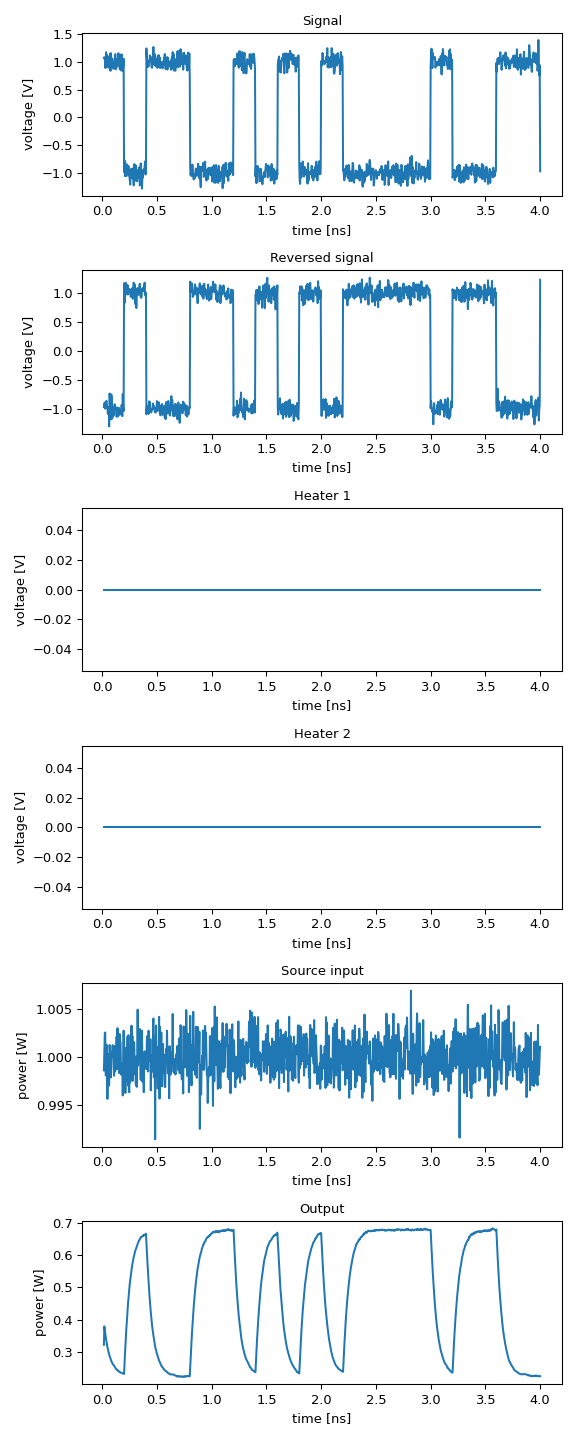### Sweeping the length of the phase shifter¶

In the following script the length of the phase shifter is swept to analyse the effect on the modulated signal. For short lengths, the modulation efficiency is dominated by the limited phase shift. For longer lengths it is limited by the RC constant of the device, due to the increased capacitance of the phase shifter.

from si_fab import all as pdk
from si_fab.components.modulator.mzm.simulate.simulate import simulate_modulation
import pylab as plt
import numpy as np
import os

# Phase Shifter
sweep_name = "sweep_length"
results_array = []
lengths = np.linspace(500, 2500, 6)
for lps in lengths:
ps = pdk.PhaseShifterWaveguide(length=lps,
trace_template=pdk.RWG450(),
junction_offset=-0.1)
vpi_lpi = 1.2
Cl = 1.1e-15  # F/um
R = 50
tau = ps.length * Cl * R
ps.CircuitModel(vpi_lpi=vpi_lpi)

# Modulator
mzm = pdk.MZMModulator(phaseshifter=ps)

results = simulate_modulation(cell=mzm,
mod_amplitude=1.0,
mod_noise=0.05,
opt_amplitude=1.0,
opt_noise=0.001,
v_heater_1=0.0,
v_heater_2=0.0,
bitrate=5e9,
n_bytes=20,
steps_per_bit=50,
center_wavelength=1.5,
simulate_sources=True,
simulate_circuit=True,
debug=False,
seed=20)
times = results["timesteps"]
results_array.append(results)

def power(t):
return np.abs(t)**2

process = [np.real, np.real, power]
fig, axs = plt.subplots(nrows=3, ncols=1, figsize=(6, 6))

for cnt, (f, pn) in enumerate(zip(process, outputs)):
for length, results in zip(lengths, results_array):
axs[cnt].set_title(pn)
axs[cnt].plot(times, f(results[pn]), label="length: {}".format(length))
if cnt == 0:
plt.tight_layout()
fig.savefig(os.path.join("{}.png".format(sweep_name)), bbox_inches='tight')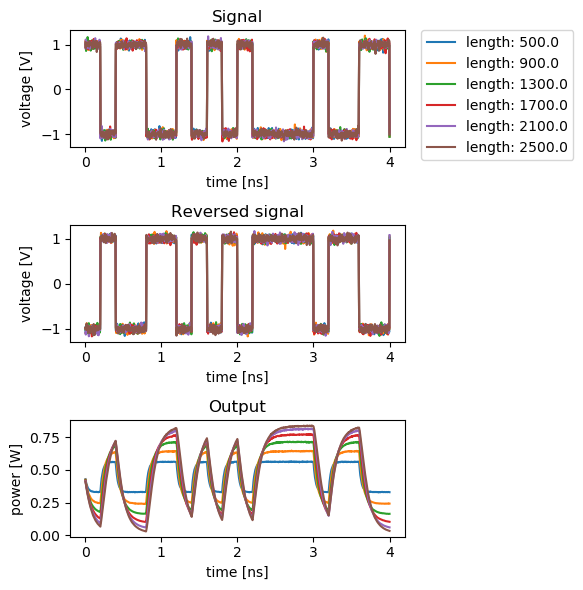### Sweeping the heater voltage¶

In the following script, the voltage applied on the heater is swept. By design, the modulation efficiency is at its maximum at 0 V. The modulation efficiency decreases as the heater voltage increases, while checking that the maximum current density is not exceeded.

from si_fab import all as pdk
from si_fab.components.modulator.mzm.simulate.simulate import simulate_modulation
from si_fab.components.heater.simulate.simulate import get_current_density
from si_fab.components.heater.pcell.cell import j_max
import pylab as plt
import numpy as np
import os

# Phase Shifter
sweep_name = "sweep_heater"
results_array = []
heater_voltages = np.linspace(0, 5.0, 6)
ps = pdk.PhaseShifterWaveguide(length=1000.0,
trace_template=pdk.RWG450(),
junction_offset=-0.1)
vpi_lpi = 1.2
Cl = 1.1e-15  # F/um
R = 50
tau = ps.length * Cl * R
ps.CircuitModel(vpi_lpi=vpi_lpi)

heater = pdk.HeatedWaveguide(name="heater")
heater.Layout(shape=[(0.0, 0.0), (100.0, 0.0)])

mzm = pdk.MZMModulator(phaseshifter=ps,
heater=heater)

sweep_name = "sweep_heater"
results_array = []
heater_voltages = np.linspace(0, 1, 6)
for v in heater_voltages:

results = simulate_modulation(cell=mzm,
mod_amplitude=1.0,
mod_noise=0.05,
opt_amplitude=1.0,
opt_noise=0.001,
v_heater_1=v,
v_heater_2=0,
bitrate=5e9,
n_bytes=20,
steps_per_bit=50,
center_wavelength=1.5,
simulate_sources=True,
simulate_circuit=True,
debug=False,
seed=20)
times = results["timesteps"]
results_array.append(results)

def power(t):
return np.abs(t)**2

process = [np.real, np.real, power, power, np.real, np.real]
fig, axs = plt.subplots(nrows=6, ncols=1, figsize=(6, 10))

for cnt, (f, pn) in enumerate(zip(process, outputs)):
for v, results in zip(heater_voltages, results_array):
axs[cnt].set_title(pn)
axs[cnt].plot(times, f(results[pn]), label="vh: {}".format(v))
if cnt == 0: